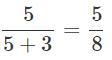Courses

# Test: Linear Equations- 3

## 20 Questions MCQ Test Mathematics (Maths) Class 8 | Test: Linear Equations- 3

Description
This mock test of Test: Linear Equations- 3 for Class 8 helps you for every Class 8 entrance exam. This contains 20 Multiple Choice Questions for Class 8 Test: Linear Equations- 3 (mcq) to study with solutions a complete question bank. The solved questions answers in this Test: Linear Equations- 3 quiz give you a good mix of easy questions and tough questions. Class 8 students definitely take this Test: Linear Equations- 3 exercise for a better result in the exam. You can find other Test: Linear Equations- 3 extra questions, long questions & short questions for Class 8 on EduRev as well by searching above.
QUESTION: 1

### David cuts a bread into two equal pieces and cuts one half into smaller pieces of equal size. Each of the small pieces is twenty grams in weight. If he has seven pieces of the bread all with him, how heavy is the original cake.

Solution:
D) 240 gm.
If she has 7 pieces totally, it means that one of those pieces is the uncut half, and the other half is cut into 6 pieces. Each of those 6 pieces has a weight of 20 gm which totally weighs 6*20= 120gm. If one half is 120 gm, then so is the other. Therefore the total weight of the cake is 120*2= 240 gm.

QUESTION: 2

### In the following number sequence, how many such even numbers are there which are exactly divisible by its immediate preceding number but not exactly divisible by its immediate following number? 3  8  4  1  5  7  2  8  3  4  8  9  3  9  4  2  1  5  8  2

Solution:

3  8  4  1  5  7  2  8  3  4  8  9  3  9  4  2  1  5  8  2

QUESTION: 3

### Mary was counting down from 34 and Thomas was counting upwards simultaneously, the number starting from 1 and he was calling out only the odd numbers. Which common number will they call out at the same time if they were calling out at the same speed?

Solution:

Mary = 34,33,32,31,30,29,28,27,26,25,24,23,22
Thomas = 01,03,05,07,09,11,13,15,17,19,21,23,25
Hence they will reach a number 23.

QUESTION: 4

Solve the given equation and find the value of y if,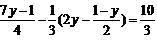Solution: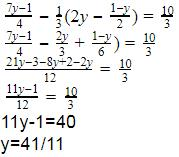QUESTION: 5

Find the value of 'm' from the expression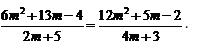Solution:
QUESTION: 6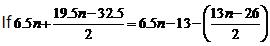then find the value of n.

Solution:
QUESTION: 7

The distance between two mile stones is 230 km and two cars start simultaneously from the milestones in opposite directions and the distance between them after three hours is 20 km. If the speed of one car is less than that of other by 10 km/h, find the speed of each car.

Solution:

Let S1 = x and S2 = x+10
Distance = speed * time
D1= 3x, D2 = 3(x+10)
Total distance = D1+D2+20
230 = 3x+30+3x+20
6x = 180
x = 30 km/h
x+10 = 40 km/h

QUESTION: 8

A store has provision which would last for a certain number of men for 21 days. For one seventh of the men it will last for how many days?

Solution:
QUESTION: 9

An MNC company employed 25 men to do the official work in 32 days. After 16 days, it employed 5 more men and work was finished one day earlier. If it had not employed additional men, it would have been behind by how many days?

Solution:

Let 1 person do 1 unit of work in 1 day
When 25 people are employed, amount of work in 1 day = 25 units
Amount of work in 16 days = 25*13 = 400
After 16 days, 5 more people are employed and work is completed in 15 days
So work done in next 15 days = 30*15 = 450
If those 5 people are not employed , No. of days needed to complete 450 units of work = 450/25 = 18
But they were supposed to complete the work by 16 days
Hence 2 extra days are needed by 25 people.

QUESTION: 10

A Bullet train left for the New York at time y minutes after x hours and reached Canada z minutes after y hour on the same day, after travelling z hrs and x minutes. How many possible values of x are there?

Solution:
QUESTION: 11

Solve for x: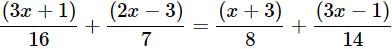Solution:

We have,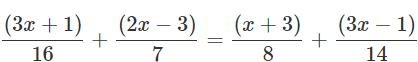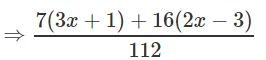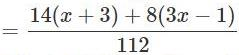⇒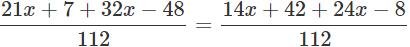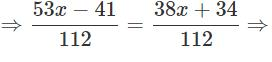553x − 41 = 38x+34 ⇒ 15x = 75

⇒ x = 75/15 = 5

QUESTION: 12

A number is 56 greater than the average of its third, quarter and one-twelfth. Find the number.

Solution:

Let the number be x. Then, One-third of x = x/3, Quarter of x = x/4
One-twelfth of x = x/12
Average of third, quarter and one-twelfth of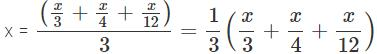According to question, we have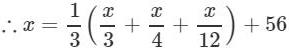⇒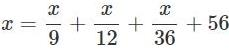⇒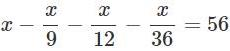⇒ 36x − 4x − 3x − x = 36 × 56 ⇒ 28x = 36 × 56
⇒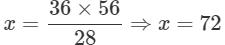Hence, the number is 72.

QUESTION: 13

If 1/3 of a number is 10 less than the original number, then the number is_____.

Solution:

Let the number bex.
According to question, we have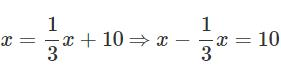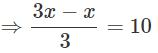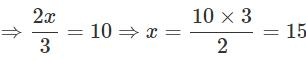Hence, the number is 15.

QUESTION: 14

Solve for x : 6(3x+2)−5(6x−1) = 6(x−3)−5(7x−6)+12x

Solution:

We have,6(3x+2)−5(6x−1)

= 6(x−3)−5(7x−6)+12x

⇒18x+12−30x+5

= 6x−18−35x+30+12x

⇒ 5x=−5 ⇒ x=−1

QUESTION: 15

The number 299 is divided into two parts in the ratio 5:8. The product of the numbers is_____.

Solution:

Ratio of two parts = 5:8

Smaller number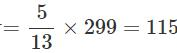Larger number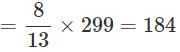So, the product of the number

= 115 × 184 = 21160

QUESTION: 16

If (2/3)rd of a number is 20 less than the original number, then the number is_____.

Solution:

Let the number be x. According to question, we have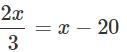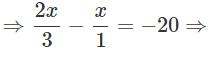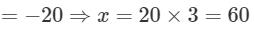QUESTION: 17

The perimeter of a rectangle is numerically equal to the area of rectangle. If width of rectangle is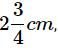then its length is_____.

Solution:

Let the length of rectangle be x cm. Width of rectangle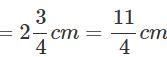∴ Area of rectangle = length × width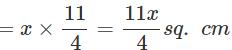Also, perimeter of rectangle = 2(length + width) Now, according to question.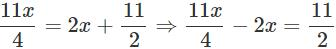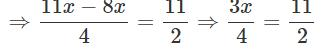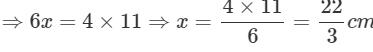QUESTION: 18

A number whose seventh part exceeds its eighth part by 1, is _____.

Solution:

Let the number be x. According to question, we have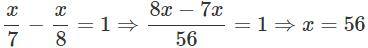QUESTION: 19

A number consists of two digits whose sum is 9. If 27 is subtracted from the original number, its digits are interchanged. Then the original number is _____.

Solution:

Let units place digit be x. So tens place digit = 9 − x.

∴ The original number = 10(9-x)+x = 90-10x + x=90 - 9x Now, after interchanging the digits, the number formed = 10 (x) + 9 - x = 9x + 9

∴ According to question, we have 90 - 9x - 27 = 9x + 9

⇒ −18x = −54 ⇒ x = 3

So, the original number

= 90−9×3 = 63

QUESTION: 20

The denominator of a rational number is greater than its numerator by 3. If 3 is subtracted from the numerator and 2 is added to its denominator, the new number becomes 1/5. The original rational number is;___.

Solution:

Let numerator be x, then denominator is x+3.

∴ The original rational number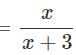Now, according to question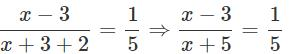On cross multiplying, we get  5(x−3 )= x+5

⇒ 5x−15 = x+5 ⇒ 5x−x = 5+15

⇒ 4x = 20 ⇒ x = 20/4 = 5

Hence, the original rational number is